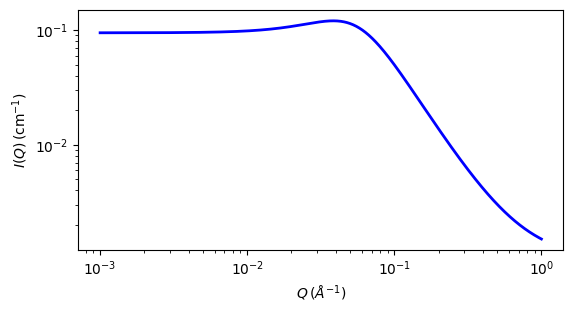# be_polyelectrolyte

Polyelectrolyte with the RPA expression derived by Borue and Erukhimovich

Parameter

Description

Units

Default value

scale

Scale factor or Volume fraction

None

1

background

Source background

cm-1

0.001

contrast_factor

Contrast factor of the polymer

barns

10

bjerrum_length

Bjerrum length

7.1

virial_param

Virial parameter

3

12

monomer_length

Monomer length

10

salt_concentration

Concentration of monovalent salt

mol/L

0

ionization_degree

Degree of ionization

None

0.05

polymer_concentration

Polymer molar concentration

mol/L

0.7

The returned value is scaled to units of cm-1 sr-1, absolute scale.

Note

Definition This model calculates the structure factor of a polyelectrolyte solution with the RPA expression derived by Borue and Erukhimovich. Note however that the fitting procedure here does not follow the notation in that reference as ‘s’ and ‘t’ are not decoupled. Instead the scattering intensity $$I(q)$$ is calculated as

$\begin{split}I(q) &= K\frac{q^2+k^2}{4\pi L_b\alpha ^2} \frac{1}{1+r_{0}^4(q^2+k^2)(q^2-12hC_a/b^2)} + \text{background} \\ k^2 &= 4\pi L_b(2C_s + \alpha C_a) \\ r_{0}^2 &= \frac{b}{\alpha \sqrt{C_a 48\pi L_b}}\end{split}$

where

$$K$$ is the contrast factor for the polymer which is defined differently than in other models and is given in barns where 1 $$barn = 10^{-24}$$ $$cm^2$$. $$K$$ is defined as:

$\begin{split}K &= a^2 \\ a &= b_p - (v_p/v_s) b_s\end{split}$

where:

• $$b_p$$ and $$b_s$$ are sum of the scattering lengths of the atoms constituting the polymer monomer and the solvent molecules, respectively.

• $$v_p$$ and $$v_s$$ are the partial molar volume of the polymer and the solvent, respectively.

• $$L_b$$ is the Bjerrum length (Å) - Note: This parameter needs to be kept constant for a given solvent and temperature!

• $$h$$ is the virial parameter (Å3) - Note: See  for the correct interpretation of this parameter. It incorporates second and third virial coefficients and can be negative.

• $$b$$ is the monomer length (Å).

• $$C_s$$ is the concentration of monovalent salt(1/Å3 - internally converted from mol/L).

• $$\alpha$$ is the degree of ionization (the ratio of charged monomers to the total number of monomers)

• $$C_a$$ is the polymer molar concentration (1/Å3 - internally converted from mol/L)

• $$\text{background}$$ is the incoherent background.

For 2D data the scattering intensity is calculated in the same way as 1D, where the $$\vec q$$ vector is defined as

$q = \sqrt{q_x^2 + q_y^2}$

Validation

As of the last revision, this code is believed to be correct. However it needs further validation and should be used with caution at this time. The history of this code goes back to a 1998 implementation. It was recently noted that in that implementation, while both the polymer concentration and salt concentration were converted from experimental units of mol/L to more dimensionally useful units of 1/Å3, only the converted version of the polymer concentration was actually being used in the calculation while the unconverted salt concentration (still in apparent units of mol/L) was being used. This was carried through to Sasmodels as used for SasView 4.1 (though the line of code converting the salt concentration to the new units was removed somewhere along the line). Simple dimensional analysis of the calculation shows that the converted salt concentration should be used, which the original code suggests was the intention, so this has now been corrected (for SasView 4.2). Once better validation has been performed this note will be removed.Fig. 97 1D plot corresponding to the default parameters of the model.

Source

be_polyelectrolyte.py

References

For further details, see , , .

Authorship and Verification

• Author: NIST IGOR/DANSE Date: pre 2010